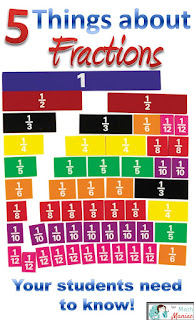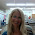## Wednesday, July 8, 2015

### Beyond Invert and Multiply Book Study Part 1

Welcome to the first installment of our Beyond Invert and Multiply book study.  You can order this book at Math Solutions or on Amazon.  There is a great video introduction to this book here if you want to learn more about it.This book is written about fraction operations and is a follow up to Beyond Pizza and Pies.  I have received several emails and Facebook messages from folks asking which book they should get if they have to pick just one.  My answer to that is that if you teach grades 2-3, go with Beyond Pizzas and Pies.  If you teach grades 4 and up you REALLY should have both.  If your students lack the foundation knowledge covered in Beyond Pizzas and Pies, many of the activities suggested in Beyond Invert and Multiply will be a struggle for your students.

### Chapter 1: Fractions As NumbersI love the way this chapter starts off with the classroom scenario of the human number line.  This is a great way to introduce kids to big ideas about number lines and doing it in a big way like this makes it very memorable.  I feel like kids really get the idea of equal spacing when doing a human number line and I think this is a great way to review what number lines are before moving into working with fractions on a number line.

The chapter lays out these 5 foundational understandings about fractions that kids need in order to compute with understanding.  These are the things that you will find your students tripping up on when learning fraction operations.  These are the things that are worth devoting some class time to before jumping into fraction operations.  They are all big conceptual ideas and together form the foundation for fraction understanding.
• Fractions are numbers and follow the same rules and properties as other rational numbers.
• The unit fraction is the building block of fractions.
• Fractions can be decomposed and recomposed in infinite ways.
• Equivalent fractions represent different ways of naming the same value.
• All rational numbers can be expressed as fractions in the form a/b, where b does not equal 0.

The other thing that really struck me in this chapter was how important number lines are.  Over and over again you see how they can help kids see fractions as numbers.  The activities and DVD clips in this section do such a great job capturing the power of the number line.  It is hard to believe that less than 10 years ago, I had never used a number line for fractions in my own mathematics or with my students.

Chapter 2: Addition and Subtraction with Fractions
This chapter dives headfirst into the research on problem types for addition and subtraction word problems.  These problem types are used with whole numbers and should also be used with fractions. If you use the Common Core for your standards, these problem types are detailed in the glossary.  I wish this table was right in the standards themselves because many people miss it.  I have included a snapshot below!  If it is out of focus or hard for you to read, you can click here to see a better copy.

If your students have never experienced these problems with whole numbers, I would be hesitant to introduce them with fractions.  I think it is worth the time to go back and do some of these problem types with whole numbers before expecting kids to do them with fractions.  When my school first started paying attention to all the different problem types, we started with the primary students on single digits, moved up to double digit computation and then finally used the different problem types with fractions.Which problem type is this?  Feel free to peek at the table above! Grab these cards here:)How would your students do on this one?  Would they use subtraction or a missing addend? Which problem type is this?

I think there is a lot of value in sitting down and writing some of each of the problem types for your students to use.  It can be challenging at first, but gets much easier with time.  If you want to explore some problems that have already been written, you can check these out!
Addition and Subtraction Facts
Double Digit Addition and Subtraction
Fraction Addition and Subtraction

There was also a chapter on the different problem types in Children's Mathematics.  You can read my thoughts on that here!

Your turn!  Please tell us how you are doing with fractions in the comments below and what you thought about this week's reading.  Has your school addressed the different problem types for addition and subtraction?  How are your students doing at developing the 5 foundational understandings for fractions?

#### 2 comments:

1.THANK YOU! Smiles and stop by anytime!

2.This really helps out! Thanks for posting!